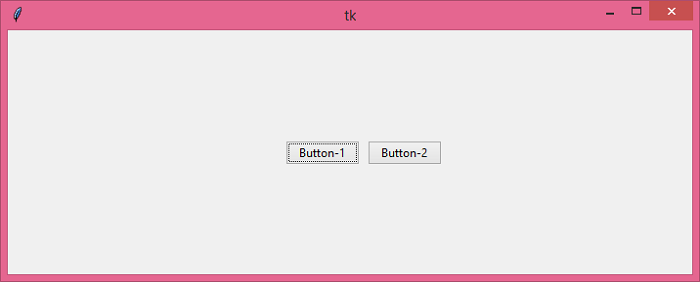# Determine which Button was pressed in Tkinter

TkinterPythonGUI-Programming

Buttons are very useful in many applications where user interaction is required. Let us suppose that we want to know which button is pressed in a given application. In order to get the information about the Button, we can use the callback function in the Button configuration. In the Callback function, we will use the print(test) function to print the button that is clicked.

## Example

#Import the required libraries
from tkinter import *
from tkinter import ttk

#Create an instance of Tkinter Frame
win = Tk()

#Set the geometry
win.geometry("700x250")

# Define function to get the information about the Button
def get_button(t):
print(t)

#Create Button Object
b1= ttk.Button(win, text= "Button-1", command= lambda t= "Button-1 Clicked": get_button(t))
b1.place(relx= .46, rely= .5, anchor= CENTER)
b2= ttk.Button(win, text= "Button-2", command= lambda t= "Button-2 Clicked": get_button(t))
b2.place(relx= .58, rely= .5, anchor= CENTER)

win.mainloop()

## Output

Running the above code will display a window with two buttons.If you click "Button-1", it will print the following on the console.

Button-1 Clicked